Courses
Courses for Kids
Free study material
Free LIVE classes
More

# Find the Common Factor## Introduction

Common factors are those factors which are common in two numbers. Factor can be a whole number, integer. Any decimal number Cannot be a Factor. We can find a common factor by individually finding the factor of two or more numbers and comparing the same factors which are present in two or more numbers, they are called common factors.

## What is a Factor?

A factor is a number that divides the other number leaving the remainder as 0. if we multiply that are factors of the product A number can have multiple numbers of factors. Let us take the example of 12. The factors of 12 are 1,2,3,4,6,12. The given number is the factor of 12 because after dividing 12 by any of these numbers the remainder will be 0. We know the very common formula that is ${\rm{dividend}} = {\rm{divisor}} \times {\rm{quotient}} + {\rm{remainder}}$. Let’s take an example of a number 12, 2 is a factor of 12 ,So 2 divide 12 Exactly and give 6 as a quotient and 0 as a reminder. 6 is also a factor of 12. So a number can have multiple number of factors like in case of 12 its factors are 1, 2, 3, 4, 6, 12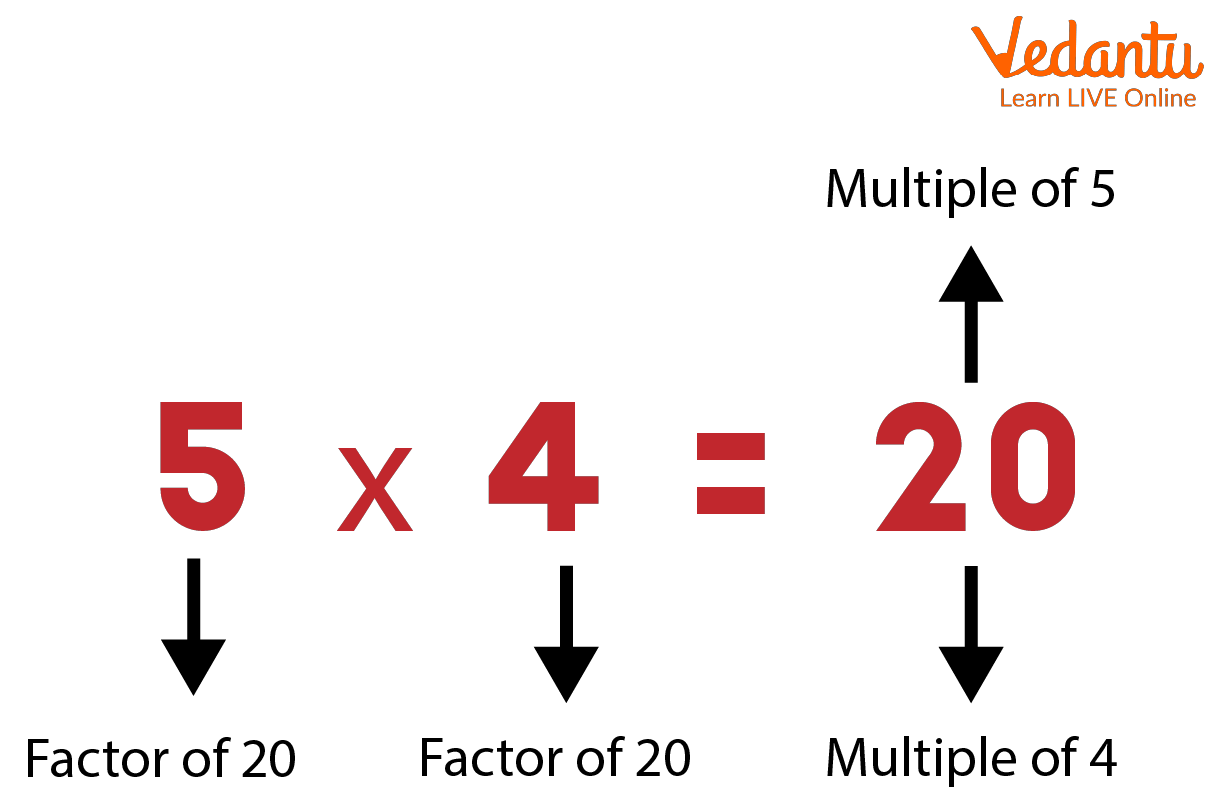Factors

## Common Factor

Those factors which are common in two numbers are called common factors. We can find out common factors of two numbers by separately finding the factor of each number and then comparing those factors,which are common to both numbers. These factors are called common factors.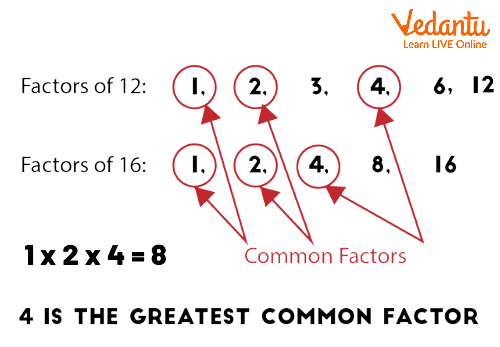Common Factor of Two Number

## Factors and its Properties:

• Finite number of factors are present for integers

• Only 0 and 1 are two numbers which do not have at least two factors.

• For a number its factor is always less than or equal to the number

• We can find out factors by multiplication and division.

## What is Prime Factorisation:

Prime factorisation of a number can be represented as a product of all the prime factors of the number; numbers involved in prime factorisation are prime numbers.ex – factor of $8 = 2 \times 2 \times 2$ so here the prime factor is 2.

Now let’s discuss the methods from which we can calculate prime factorisation:

• Firstly we have to divide given number to the smallest prime number

• Repeat the process again by dividing quotient of the above solution by the smallest prime number

• We have to repeat this process again and again until the quotient becomes 1.

• Then multiply all the prime factors we get from various steps ,the result of that multiplication is called prime factorisation.

## What is HCF

HCF is the highest common factor as its name suggests it is the greatest common factor of two or more numbers. Highest common factor is the largest number which can divide both the numbers. Let’s take an example, let a and b are two numbers so the HCF of two numbers is the largest number which can simultaneously divide both a and b. HCF is also called GCF– Greatest common factor.

Example-let’s take two number 12 and 36

Factors of 12–1,2,3,4,6,12

Factors of 36–1, 2,3,4,6,9,12,18,36

The common factor of 12 and 36 are 1,2,3,4,6,12

And the highest common factor of two numbers is 12.

## What is LCM

LCM is the least common multiple as its name suggests it is the lowest number that can be divisible by both numbers. Let’s understand this with the help of an example – take two numbers 3 and 5. Multiples are the number that divided by another number will leave the remainder as 0.

Multiples of 3–3,6,9,12,15,18,21,24,27,30

Multiples of 5–5,10,15,20,25,30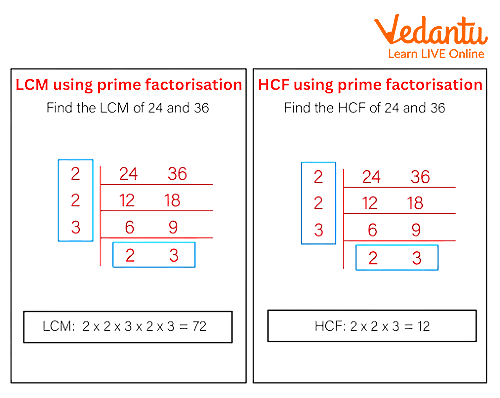Image Showing Lcm and Hcf of Two Numbers

• There are finite number of factors of a given number

• Every number is a factor of itself

• Every number has one common factor that is 1

• Factor is exact divisor of a number

## Solved Questions:

1.Find the prime factors of 30.

We know prime numbers are those numbers which are either multiple of 1 or the number itself.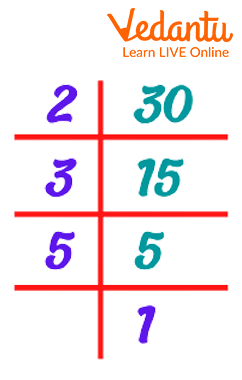So prime factors of 30 are 2,3,5

2. Find the common factor of 5 and 30.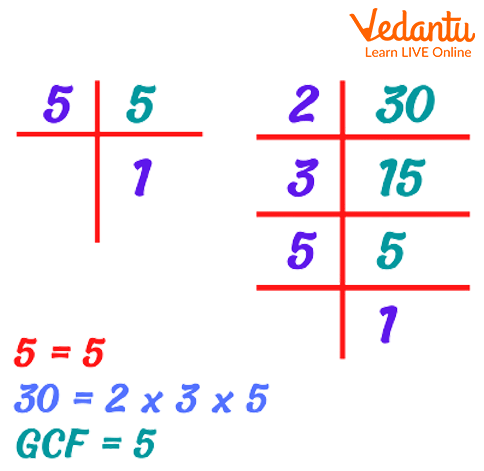GC of 5 and 30

So from matching factors of both 5 and 30 we get the common factor 5

## Conclusion:

Here in this article we learned about common factors and how to find out common factors as The name itself suggests that there is a common number which divides two numbers exactly. In this article we also learned about factors, HCF, LCM, prime factorisation and also learnt some interesting facts about factors.

Last updated date: 24th Sep 2023
Total views: 69.3k
Views today: 1.69k

## FAQs on Find the Common Factor

1. Can factors be infinite?

Factors can’t be infinite. Each and every number has finite factors.

2. Expand GCF ?

Greatest Common Factor

3. What is the property of HCF?

• HCF of any two or more numbers is never greater than any of the given numbers.

• The HCF of two or more prime numbers is always 1.